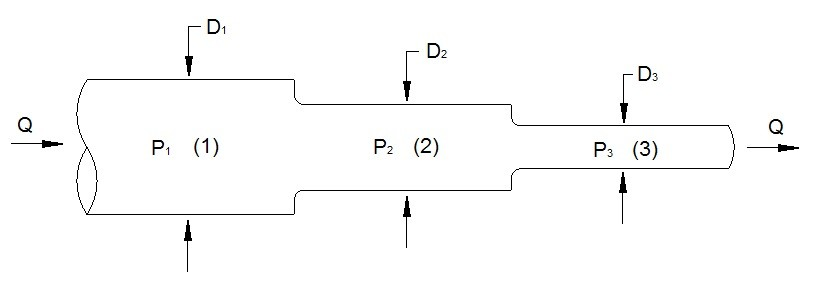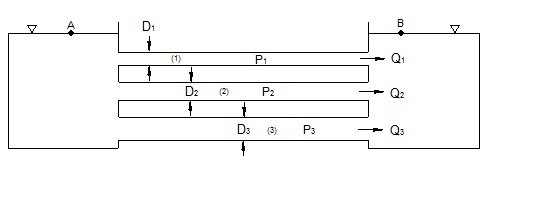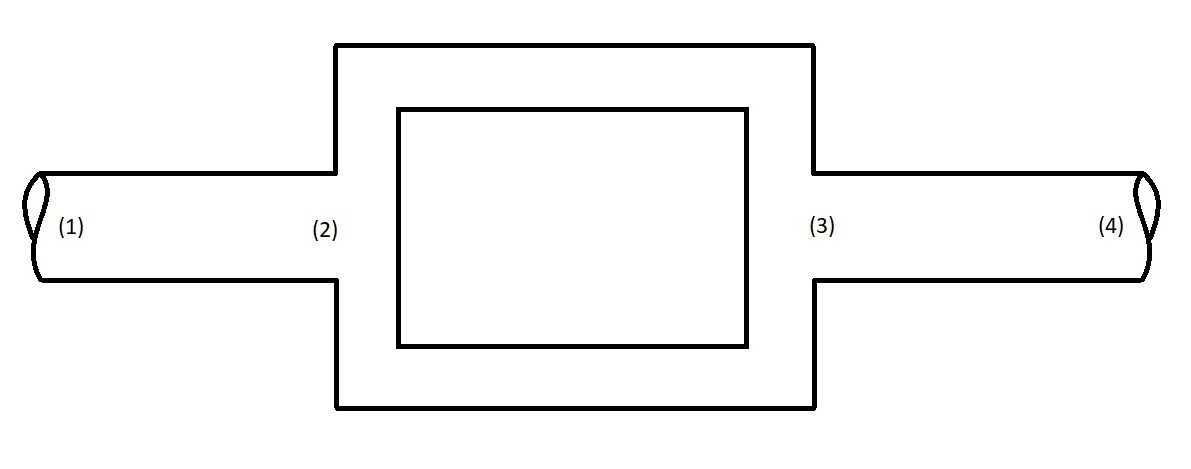# Multiple Pipe Systems

Most pipe systems are a multiple pipe systems.  Multiple pipe systems can have pipes that are running in parallel to one another as well as in series with each other, or a combination of both.  For simplicity, I am going to discuss simple multiple pipe systems.

### Multiple Pipe Systems in Series

First, let’s take a look at pipe’s that are in series.  Below is an image that is shows three pipes with different cross-sections in series.When a set of pipes are in series the flow rate through those pipes will be constant.

(Eq 1) $Q_1=Q_2=Q_3$

However, the head loss caused by each pipe will be different.  As result, the total head loss will be a product of the three head losses.

(Eq 2)  $h_{L_{tot}}=h_{L_1}+h_{L_2}+h_{L_3}$

### Multiple Pipe Systems in Parallel

In addition to flow running in series, it can also run in parallel.  Bellow is a example of three pipes that are in parallel.For a flow that is running in parallel each pipe will have different flow rate.  Because of this the total flowrate of the system of pipe will be a product the individual flow rates.

(Eq 3) $Q=Q_1+Q_2+Q_3$

The head loss, on the other hand, will be the same across each pipe.

(Eq 4) $h_{L_1}=h_{L_2}=h_{L_1}$

### Pipes in Series and in Parallel

In reality a system of pipes could be a combination of the pipes in series and in parallel. The image below shows a simple case.In this image the two center pipe between points 2 and 3 are in parallel with each other.  However, they are in series with pipe 1-2 and pipe 3-4.  Hence, depending on what is of interest will determine how you would solve this problem.  For example, if you were interested in the head loss from point 1 to 4 than you would need to add the head loss of pipe 1-2 and pipe 3-4 to one of the pipes between points 2-3 to find the total head loss. While if you were interested in the flow rate of the hole system you would only need to determine the flowrate of either pipe 1-2 or pipe 1-3.  However, since the pipes between points 2 and 3 are in parallel the total system flow rate will be split between the two in a certain ratio where the flow will favor the pipe that has the least resistance.

Previous

|